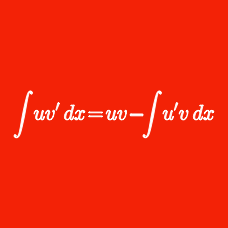Calculus

# Integration U-substitution - Given U

Using the substitution $$\ln x=t,$$ which of the following is equal to $\int_{e}^{e^{6}} \frac{3(\ln x)^2}{x} dx ?$

Evaluate $$\displaystyle{\int_0^{3}\frac{12x+6}{x^2+x+1}dx.}$$

Using the substitution $$u=\sin x,$$ evaluate $$\displaystyle{\int_0^\frac{\pi}{2}\sin^{10} x\cos xdx.}$$

Evaluate $$\displaystyle{\int_0^\frac{\pi}{32}3\tan8xdx.}$$

Using the substitution $$u=e^{x^2},$$ evaluate $$\displaystyle{\int_0^{3}12x e^{x^2}dx.}$$

×